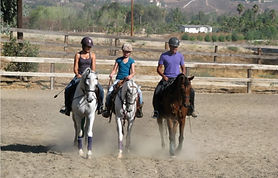top of page## LESSONS

One hour Group or Private
(Group or private lessons given at the discretion of the instructor)

\$55.00 each
(when pre paid in a package of four lessons to be used in a one month period)

\$65.00 each
(when scheduled lesson to lesson)

½ hour lessons

(Group or private lessons given at the discretion of the instructor)

\$35.00 each
(when prepaid in a package of four lessons to be used in a one month period)

\$45.00 each
(when scheduled lesson to lesson)bottom of page